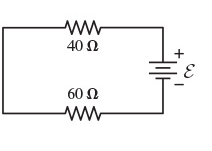# Problem: Suppose that ℰ = 65 V. (Figure 1)What is the potential difference across the 40 Ω resistor? Express your answer with the appropriate units.What is the potential difference across the 60 Ω resistor?

###### FREE Expert Solution

Ohm's law:

$\overline{){\mathbf{V}}{\mathbf{=}}{\mathbf{i}}{\mathbf{R}}}$

Equivalent resistance for series resistors:

$\overline{){{\mathbf{R}}}_{\mathbf{e}\mathbf{q}}{\mathbf{=}}{{\mathbf{R}}}_{{\mathbf{1}}}{\mathbf{+}}{{\mathbf{R}}}_{{\mathbf{2}}}}$

(a)

Req = R40 + R60 = 40 + 60 = 100Ω

icircuit = ε/Req = 65/100 = 0.65 A###### Problem Details

Suppose that ℰ = 65 V. (Figure 1)

What is the potential difference across the 40 Ω resistor? Express your answer with the appropriate units.

What is the potential difference across the 60 Ω resistor?